# TriMesh#

Title
TriMesh Element
Dependencies
Bokeh
Backends
Matplotlib
Bokeh
```import numpy as np
import holoviews as hv

from holoviews import opts
from scipy.spatial import Delaunay

hv.extension('matplotlib')
```A `TriMesh` represents a mesh of triangles represented as the simplexes and vertices. The simplexes represent the indices into the vertex data, made up of three indices per triangle. The mesh therefore follows a datastructure very similar to a graph, with the abstract connectivity between nodes stored on the `TriMesh` element itself, the node or vertex positions stored on a `Nodes` element and the concrete `EdgePaths` making up each triangle generated when required by accessing the edgepaths attribute.

Unlike a Graph each simplex is represented as the node indices of the three corners of each triangle rather than the usual source and target node.

We will begin with a simple random mesh, generated by sampling some random integers and then applying Delaunay triangulation, which is available in SciPy. We can then construct the `TriMesh` by passing it the simplexes and the vertices (or nodes).

```n_verts = 100
pts = np.random.randint(1, n_verts, (n_verts, 2))
tris = Delaunay(pts)

trimesh = hv.TriMesh((tris.simplices, pts))
trimesh
```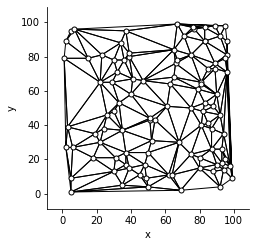To make this easier TriMesh also provides a convenient `from_vertices` method, which will apply the Delaunay triangulation and construct the `TriMesh` for us:

```hv.TriMesh.from_vertices(np.random.randn(100, 2))
```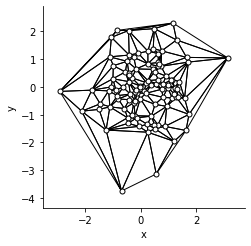Just like the `Graph` element we can access the `Nodes` and `EdgePaths` via the `.nodes` and `.edgepaths` attributes respectively.

```trimesh.nodes + trimesh.edgepaths
```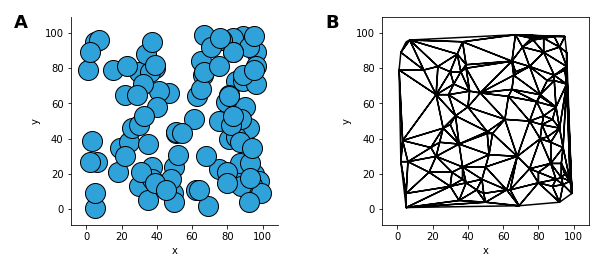Now let’s make a slightly more interesting example by generating a more complex geometry. Here we will compute a geometry, then apply Delaunay triangulation again and finally apply a mask to drop nodes in the center.

```# First create the x and y coordinates of the points.
n_angles = 36

angles = np.linspace(0, 2*np.pi, n_angles, endpoint=False)
angles = np.repeat(angles[..., np.newaxis], n_radii, axis=1)
angles[:, 1::2] += np.pi/n_angles

nodes = np.column_stack([x, y, z])

# Apply Delaunay triangulation
delaunay = Delaunay(np.column_stack([x, y]))

xmid = x[delaunay.simplices].mean(axis=1)
ymid = y[delaunay.simplices].mean(axis=1)
```

Once again we can simply supply the simplices and nodes to the `TriMesh`.

```hv.TriMesh((simplices, nodes))
```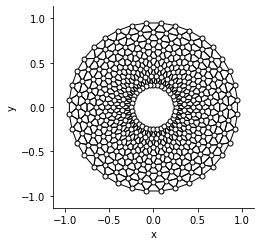We can also do something more interesting, e.g. by adding a value dimension to the vertices and coloring the edges by the vertex averaged value using the `edge_color` plot option:

```hv.TriMesh((simplices, hv.Points(nodes, vdims='z'))).opts(
opts.TriMesh(cmap='viridis', edge_color='z', filled=True, fig_size=200))
```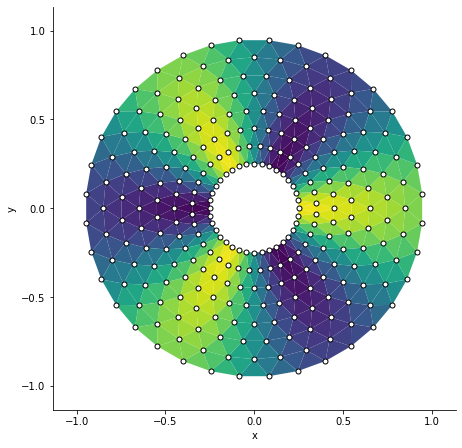For full documentation and the available style and plot options, use `hv.help(hv.TriMesh).`

This web page was generated from a Jupyter notebook and not all interactivity will work on this website. Right click to download and run locally for full Python-backed interactivity.